Next: Conditioning Up: No Title Previous: Patriot Missile Software

# Backward and Forward Errors

Suppose that an approximation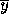to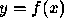is computed in an arithmetic of precision, where f is a real scalar function of a real scalar variable. How should we measure the ``quality" of?

In many cases, we would be happy with a tiny relative error,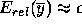, but this cannot always be achieved. Instead of focusing on the relative error ofwe can ask ``for what set of data have we actually solved our problem?", that is, for what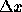do we have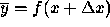? In general, there may be many such, so we should ask for the smallest one. The value of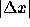, possibly divided by |x|, is called the backward error. The absolute and relative errors ofare called forward errors. Figure 3 highlights the relationship between these errors.

The process of bounding the backward error of a computed solution is called backward error analysis. It interprets rounding errors as being equivalent to perturbations in the data. The data frequently contains uncertainties due to previous computations or errors committed in storing numbers on the computer. If the backward error is no larger than these uncertainties then the computed solution can hardly be criticized.

A method for computingis called backward stable if, for any x, it produces a computedwith a small backward error, that is,for some small. The definition of ``small" is context dependent. Many a times we use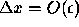, whereis the machine epsilon.

Dinesh Manocha
Wed Jan 8 00:43:08 EST 1997